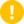## Name

ST_Equals — Returns true if two geometries include the same set of points.

## Synopsis

`boolean ST_Equals(`geometry A, geometry B`)`;

## Description

Returns TRUE if the given Geometries are "spatially equal". Use this for a 'better' answer than '='. Note by spatially equal we mean ST_Within(A,B) = true and ST_Within(B,A) = true and also mean ordering of points can be different but represent the same geometry structure. To verify the order of points is consistent, use ST_OrderingEquals (it must be noted ST_OrderingEquals is a little more stringent than simply verifying order of points are the same).This function will return false if either geometry is invalid except in the case where they are binary equal.Enhanced: 3.0.0 enabled support for `GEOMETRYCOLLECTION`This method implements the OGC Simple Features Implementation Specification for SQL 1.1. s2.1.1.2This method implements the SQL/MM specification. SQL-MM 3: 5.1.24

Changed: 2.2.0 Returns true even for invalid geometries if they are binary equal

## Examples

```SELECT ST_Equals(ST_GeomFromText('LINESTRING(0 0, 10 10)'),
ST_GeomFromText('LINESTRING(0 0, 5 5, 10 10)'));
st_equals
-----------
t
(1 row)

SELECT ST_Equals(ST_Reverse(ST_GeomFromText('LINESTRING(0 0, 10 10)')),
ST_GeomFromText('LINESTRING(0 0, 5 5, 10 10)'));
st_equals
-----------
t
(1 row)
```